Home / DC Machines / DC Generator: Types, Working, Parts | Classification of DC Generators

# DC Generator: Types, Working, Parts | Classification of DC Generators

Want create site? Find Free WordPress Themes and plugins.

When a conductor is moved through a magnetic field, a voltage is induced across the conductor by electromagnetic induction. Electromagnetic induction is the fundamental principle underlying electrical generators. A DC generator is one of the simplest generators to understand, so it is introduced first.

## Classification of DC Generators

Several types of DC generators are discussed in this section. The most basic DC generator is a permanent magnet type that is commonly used in small applications.

Industrial generators use electromagnets to generate the required magnetic field. These generators can be classified according to how the magnetic field is generated.

Figure 1 shows an overview of this classification method; each type of generator is discussed in this section.Figure 1 Classification of DC Generators by Electromagnetic Field Windings

## DC Generator Working Principle

Figure 2 shows a greatly simplified view of a DC generator. It consists of a single loop of wire that rotates in a magnetic field. Keep in mind that practical DC have many turns wrapped on an iron core.

For simplicity, a permanent magnet is shown for the field. Notice that each end of the loop is connected to a split-ring arrangement. This conductive metal ring is called a commutator; it is a rotary electrical switch that periodically changes the direction of current from the rotor to the external circuit.

As the wire loop rotates in the magnetic field, the split commutator ring also rotates. Each half of the split ring rubs against the fixed contacts, called brushes, which are carbon blocks that press against the rotor of a motor or generator to make an electrical connection between the fixed part and the rotating part. The brushes connect the rotating wire to the external circuit.

Driven by an external mechanical force, the loop of wire rotates through the magnetic field and cuts through the flux lines at varying angles, as illustrated in Figure 3. At position A in its rotation, the loop is effectively moving parallel with the magnetic field. Therefore, at this instant, the rate at which it is cutting through the magnetic flux lines is zero.

As the loop moves from position A to position B, it cuts through the flux lines at an increasing rate.

At position B, it is effectively moving perpendicular to the magnetic field and is thus cutting through a maximum number of lines.

As the loop rotates from position B to position C, the rate at which it cuts the flux lines decreases to a minimum (zero) at C.

From position C to position D, the rate at which the loop cuts the flux lines increases to a maximum at D and then to a minimum again at A.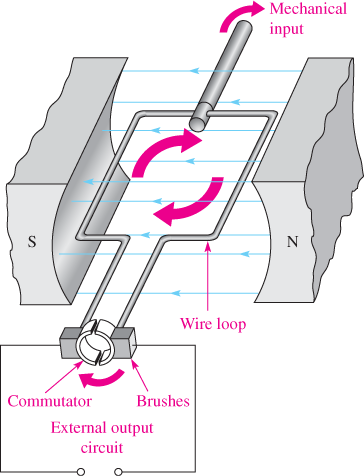Figure 2 Basic DC Generator

As you know, when a wire cuts magnetic field lines, a voltage is induced. The amount of induced voltage is proportional to the number of loops (turns) in the wire and the rate at which it is moving with respect to the magnetic field.

The angle at which the wire moves with respect to the magnetic flux lines determines the amount of induced voltage because the rate at which the wire cuts through the flux lines depends on the angle of motion.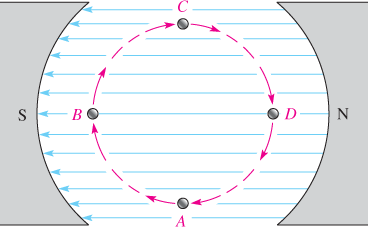Figure 3 End View of Loop Cutting Through the Magnetic Field

Figure 4 illustrates how a voltage is induced in the external circuit as a single loop of wire rotates in the magnetic field.

Assume that the loop starts in the instantaneous vertical position (position A), so the induced voltage is zero.

As the loop rotates, the induced voltage builds up to a maximum at position B, as shown in the figure.

Then, as the loop continues from B to C, the voltage decreases to zero at C where it is back to zero.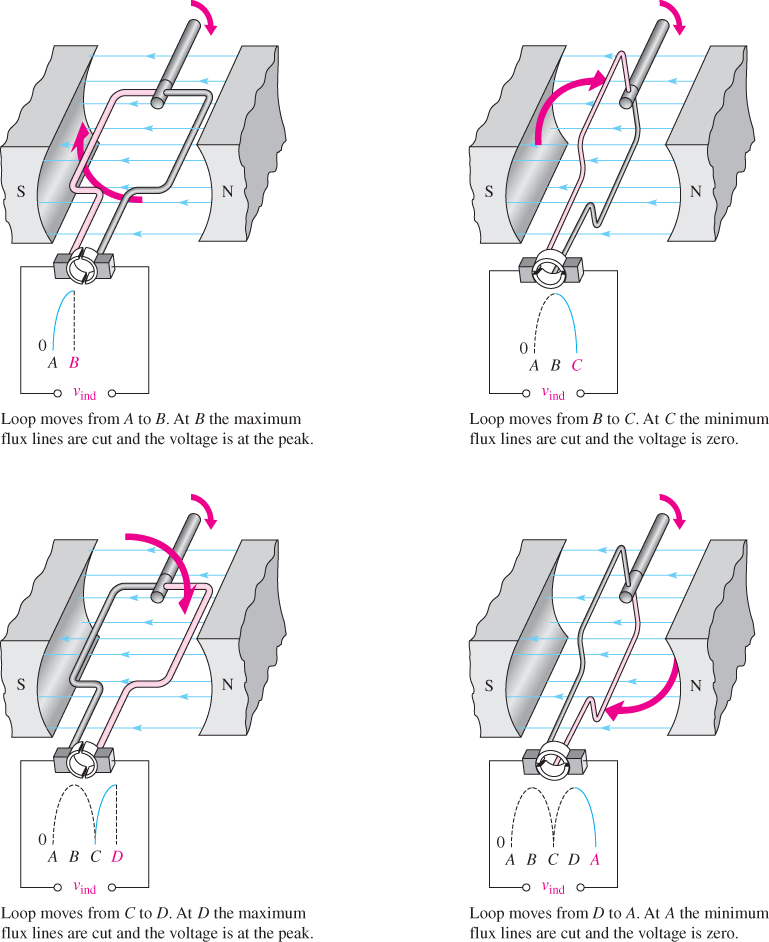Figure 4 Basic Operation of a DC Generator

During the second half of the revolution, the brushes switch to opposite commutator sections, so the polarity of the voltage remains the same across the output. Thus, as the loop rotates from position C to D and then back to A, the voltage increases from zero at C to a maximum at D, and back to zero at A.

Figure 5 shows how the induced voltage varies as a single wire loop in a DC generator goes through several rotations (three in this case). This voltage is DC because the polarity does not change. However, the voltage is pulsating between zero and its maximum value.

Figure 6 shows how extra loops produce extra segments of voltage that smooth out the ripple and raise the average voltage rating.

Each additional loop added to the generator ensures that the DC voltage level remains close to the peak voltage. Capacitors can also be used as filters to help smooth out the pulsing DC ripple.Figure 5 Induced Voltage over Three Rotations of the Loop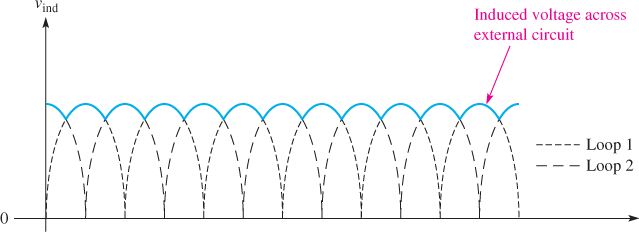Figure 6 Induced Voltage for a Two-Loop Generator. The second loop doubles the number of segments, which causes less variation in the induced voltage going from peak to zero.

## Calculating Induced Voltage

The following equation calculates the amount of induced voltage that a wire loop can produce as it moves through a magnetic field.

The values are flux density, measured in webers per square meter; the length of the conductor exposed to the field, measured in meters; and the velocity of the conductor, measured in meters per second.

${{V}_{ind}}=Blv$

Where

Vind = induced voltage, in V

B = flux density that is perpendicular to the motion, in Wb/m2

l = length of the conductor, in m

v = velocity of the conductor, in m/s

## DC Generator Parts

Figure 7 shows a small industrial DC generator that was taken out of service and disassembled.

The DC generator is divided into two parts: the moving portion called the rotor (which includes the fan, armature, commutator, and shaft [shown in the figure]) and a stationary part called the stator (which includes the end plates and case [shown in the figure] and the stator windings [not shown in the figure]).

The term armature in a generator or a motor refers to the winding in which a voltage is generated by virtue of its relative motion with respect to a magnetic flux field.

The armature can be on either the rotor or the stator; the key is relative motion. The energy to rotate the rotor can come from any mechanical energy source, such as a wind turbine or a geothermal steam turbine.

The voltage produced by a DC generator is used to charge batteries, or the DC power can be sent through a power electronic frequency converter (PEFC) or electronic inverter, where it is turned into AC. In large systems, the ac can be interfaced with the power grid.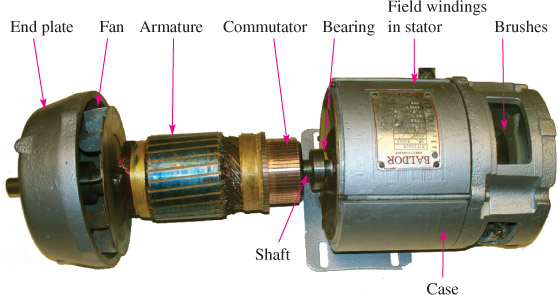Figure 7 Industrial DC Generator. The brushes are shown in Figure 8, and the field windings are shown in Figure 9

Rotor

In a DC generator, the rotor assembly includes the shaft and armature coils, which are the coils that produce power in a motor or generator.

In practical generators, multiple coils are pressed into slots in a ferromagnetic-core rotor assembly.

The armature coils are connected to the commutator, which is a rotating switch that connects coils through brushes to the output terminals.

The entire assembly rotates in the magnetic field. The fan blades on the end of the shaft ensure that sufficient air moves through the generator to keep it cool when the armature is rotating. The shaft is supported by a bearing on each end.

## Brushes and Commutator

Most generators have a number of rotor coils, and the commutator constantly switches different coils to the output.

Commutation is the provision of a unidirectional current from the generator as different coils are switched to the output. Thus, one of the brushes always has positive voltage potential, and the other always has a negative potential. In effect, the commutator and brushes serve as a mechanical rectifier.

The commutator is divided into segments, with each pair of segments connected to the end of a coil.

The voltage from several coils is combined because the brushes can contact more than one of the commutator segments at once.

The loops are sequentially connected to the output when they are near maximum voltage, so the pulsating output voltage is much smoother than is the case with only one coil or loop, as shown previously.

Figure 8a shows a close-up view of how the ends of each coil on the armature are soldered to a commutator segment. A single carbon brush is also shown in this image to show the relative size.

Two carbon brushes—one on each side of the commutator—are used to make contact between the commutator and the output terminals. All of the output currents must pass through these brushes.

Figure 8b shows a brush mounted in place against the commutator. A very strong spring holds the brushes against the commutator to maintain a low-resistance connection.

Carbon brushes wear down eventually because of the contact with the commutator and because of electrical arcing.

When the brush is worn down, the spring can be pulled back and a new brush can be placed into the holder.

Figure 8 Commutator and Brush

Stator

Recall that that the stationary part of a generator is called the stator. Figure 9 shows the stator with the rotor removed.

The rotor fits inside the stator, which is the stationary part of the generator that has the field windings mounted onto a steel core.

Notice that the core is shaped to follow the rotor’s curvature and thus keep the air gap to a minimum. The core, case, rotor, and air gap are all part of the magnetic circuit for the stator.

The windings in the stator are connected to an external voltage source, which causes the current in the stator coils, thus creating the strong magnetic field that is directed across the air gap and into the rotor.

As the armature rotates from an external energy source, the armature coils cross the magnetic field lines from the stator and voltage is induced across the armature coils.

A coiled spring is used to hold tension on the carbon brush in a generator. The spring provides enough pressure to ensure that the brush makes electrical contact with the commutator segments.

The brush must be checked periodically to ensure that it has not worn down too much. Brushes are the main component of a generator that must be checked for wear and replaced over time.

Slip rings should be checked at the same time to look for signs of scratching or wear.Figure 9 Stator AssemblyFigure 10 Spring for a Carbon Brush

## Separately Excited and Self-Excited Generators

DC generators are classified by how they obtain current for the field electromagnets (called excitation current) and how they are wired. We will look first at excitation methods.

As you have seen, nearly all DC generators use an electromagnet to create the required magnetic field (very small generators can use a permanent magnet).

An electromagnet requires current to produce the field (except for a small residual field). Current can be provided for the field in one of two ways: (1) use a separate source for field current or (2) use a portion of the generator’s own output to supply current for the field. Both methods have advantages and disadvantages, which will be discussed later in this section.

## Series, Shunt, and Compound DC Generators

The second factor in classifying DC generators is how the field and armature coils are wired for a self-excited generator.

They can be wired in series, shunt, or compound generators. The term shunt means “parallel,” and in the case of generators, this means that the field coils are in parallel with the armature coils.

Keep in mind that schematics generally show a single field coil, but it is split to put part of it on each pole of the magnetic assembly.

## Series DC Generators

In a series-wound DC generator, the field windings are in series with the armature windings and the load.

Because of the series connection, the field current, armature current, and load current are all identical.

If the load increases (less resistance), current increases; hence the field current and magnetic field increase. The result is increased output voltage for the increased load current, which tends to raise the output current even more.

This is classic positive feedback, an undesirable situation for most applications. For this reason, the series-wound DC generator has only limited applications.

## Shunt Generators

A shunt generator is one where the field winding and armature winding are in parallel.

Unlike the series-wound generator, current in the field windings is not part of the load current. However, there is still a loading effect on a DC shunt generator.

When the output current increases, more voltage is dropped across the resistance of the armature coil, causing the output to decrease. Another effect that reduces the output is called armature reaction.

Armature reaction is an effect caused by armature current whereby the current in the armature generates an mmf that tends to distort and decrease the magnetic flux from the field coils.

The decrease from these two effects means that a self-excited generator supplies less current to the field windings, which reduces the magnetic field and reinforces the tendency for the voltage to drop under load.

## Compound Generators

A compound generator has two field windings: one in series and one in parallel.

The load current and the series field winding are the same, so an increase in load current tends to increase the magnetic field from the series winding and hence the output tries to increase.

The increase in load current in the shunt winding tends to compensate for this by decreasing the output.

The series and shunt windings combine and tend to keep the magnetic field strength relatively constant, so the output voltage tends to be independent of the load in the typical case; this is called a flat compounded generator.

The compensation can keep the output to a 1% to 2% variation over the full range of loads. Compound generators have the most consistent output for varying loads of any of the three types.

## Separately Excited DC Generator

Figure 11 shows a diagram of a separately excited shunt generator. Notice that the armature is represented as a circle and a brush is shown on each side of the armature.

The two wires connected to the brushes are identified as A1 and A2 to indicate that they are armature wires and this is where electrical power is removed for the load.

Recall that separate excitation means that there is an external supply for the field current. In Figure 11, the field voltage comes from a battery and is connected to terminals labeled F1 and F2.

A rheostat (a type of variable resistor that controls current) is shown to adjust the field current, but this is usually some form of electronic control. This armature supplies current directly to the load and the field does not share load current.

With no load, the output voltage is the same as the armature voltage. When a load is connected, the output drops because of an IR drop in the armature and because armature reaction, as described for shunt generators, which tends to shift the magnetic field due to armature current.Figure 11 Separately Excited DC Generator. The separately excited dc generator has the field voltage connected to an external dc source.

A separately excited generator is controlled by varying the source current, which can be useful in renewable energy systems such as wind turbines in which the shaft speed may vary.

Controlling the excitation can help maintain a constant DC output. The disadvantage of this method is that a separate source of DC must be available, which adds to the cost.

## Self-Excited Series DC Generator

A self-excited generator is one in which some of the output is used to provide current for the field.

The self-excited series DC generator has the field winding in series with the armature; thus, the armature current, field current, and load current are the same. Figure 12 shows the arrangement.

When the generator is started, a small residual magnetism produces a field and the output is small. As current is supplied to the load, the increased current causes the output voltage to increase. Thus, the voltage varies with load current, an undesirable characteristic for generators.

Although a series-wound DC generator has a very limited application by itself, series field coils are very useful in compound generators, as you will see.

The generator starts from residual magnetism in the stator’s poles. This is a case where a small amount of hysteresis is an advantage because the core “remembers” the last state it was in and begins to output a small voltage.

If the generator has not been used for a long time, it may be necessary to apply an external source to the field windings to start it; this is called flashing the fields.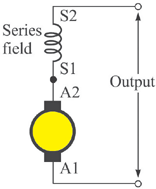Figure 12 Self-Excited Series DC Generator. The self-excited series dc generator has the field winding in series with the armature and load (not shown).

Most DC generators are self-excited because the method is simpler; they use the residual magnetism in their fields to produce the required field at start-up.

Once the voltage is produced, the self-excited generator can tap some portion of the output current to control the generator’s output. The voltage regulation tends to be less efficient than that found in separately excited generators.

## Self-Excited DC Shunt Generator

The majority of DC generators are self-excited shunt generators. A self-excited shunt generator is a generator that supplies voltage from its armature to create the field current. The field and armature windings are parallel. Figure 13 shows the configuration.

In the schematic shown, a rheostat (R1) is shown in series with the shunt field to control the current in the field. This connection allows a small fraction of armature current to be routed back through the field winding to create the magnetic field.

The amount of power the field needs is usually less than 2% of the generator’s output, and the rheostat is set for the required amount.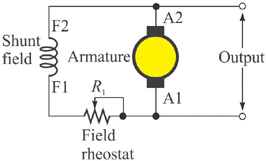Figure 13 Self-Excited Shunt Generator. The self-excited shunt dc generator has the field winding and field rheostat in parallel with the armature and load (not shown).

The field winding has a fixed resistance, so the field current is proportional to the applied voltage. An increase in field current increases the magnetic field up to the point at which core saturation occurs.

Saturation is the point where an increase in magnetic field intensity (H) in a core does not result in an increase in flux density.

The rheostat can control the output voltage by adjusting the field current up to the point of saturation.

When a self-excited shunt generator is turned at a constant speed, the output voltage remains fairly constant.

Because the field is connected in parallel with the armature, the voltage applied to the field also remains fairly constant. This equilibrium continues as long as the load is constant and it remains at a constant speed.

If the voltage is used for charging a bank of batteries, a small change in voltage is not a problem.

## Long-Shunt and Short-Shunt Compound Generators

As you have learned, a flat compounded generator uses both a series field and a shunt field to maintain nearly constant output voltage over a range of loads.

The series field consists of a few turns of very large wire and it is connected in series with the armature, whereas the shunt field consists of many turns of smaller-diameter wire.

The shunt field coil can be connected in one of two ways. The first is to connect field coils, armature, and rheostat, as shown in Figure 14 with the connections labeled.

In this configuration, the combination of the shunt field coil and its series rheostat across are both in parallel with the combination of the armature coil and the series field coil. This configuration is called a long-shunt generator.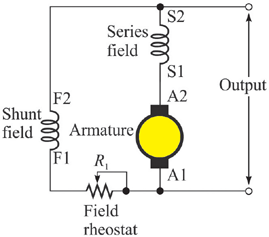Figure 14 Long-Shunt Compound Generator

The second way to connect the coils is to connect the circuit shown in Figure 15. In this configuration, the combination of the shunt field with its series rheostat is connected across the armature only. This arrangement is called a short-shunt generator.

In both the long-shunt and short-shunt configurations, the coils can be wound on the same pole pieces.Figure 15 Short-Shunt Compound Generator

## Controlling the Output Voltage

The output voltage of a DC generator can be controlled in one of two ways.

A prime mover is the source of kinetic energy that is converted to electrical energy by the generator; it can be a steam turbine, a hydroelectric generator, a wind turbine, or another source.

If the prime mover rotates at constant speed, the field current control is the method that is used to vary the output.

The first method is to maintain a constant rotational speed and vary the field current to vary the output voltage. The output voltage follows the field current before saturation.

The second method is to vary the rotational speed of the generator. The output voltage is proportional to the rotational speed.

Frequently, a renewable energy system does not have a consistent rotational speed (some wind turbines, for example) but needs to have a constant output voltage. In this case, the field current can compensate for speed changes.

The field current can be controlled automatically by an electrical circuit called a voltage regulator.

A voltage regulator is an electrical circuit that automatically maintains a constant output voltage level over variations in load.

If the voltage regulator uses solid-state devices such as insulated gate bipolar transistors (IGBTs) or operational amplifiers (op-amps), the voltage can be controlled automatically, even as the load changes or the speed changes.

If the voltage regulator is an older style, you may need to adjust the field current manually to set the voltage to the level that the application requires.

## Polarity in DC Generators

The polarity of a DC generator can be reversed in one of two ways.

First, the direction of rotation can be changed so the polarity of the armature voltage is reversed. If the generator is driven by a wind turbine or steam turbine, this may not be an option.

Second, the polarity of the field winding with respect to the armature winding is reversed. In some cases, you will have trouble getting the polarity the way you want it and you may need to flash the field with an external voltage to change the polarity.

(Flashing the field restores a small amount of residual magnetism in the core by briefly connecting a low voltage external source to the field connections when the field has been isolated.)

Another way to get the polarity correct to the load is to reverse the wires from the load that are connected to the + and − terminals on the generator. The net result is the same, but reversing the load does not change the polarity of the generator.

Example

You need to adjust the output voltage of a DC generator.

• Explain the two ways you can change the output voltage of the generator.
• Describe two ways you can change the polarity of the voltage that is sent to the load if you could not reverse the direction of rotation.

Solution

• One way is to change the speed of the armature if possible. The second way is to adjust the voltage regulator.
• One way to change the polarity is to reverse the polarity of the field winding. the second way is to change the polarity of the armature terminals where the load is connected.

Review Questions

1. How is the induced voltage created?
2. Explain the parts of a simple DC generator.
3. What is the function of the armature in a DC generator?
4. What is the function of the field windings in a DC generator?
5. What type of DC generator tends to have a constant voltage regardless of the load?

Answers

1. By relative motion of a conductor to a magnetic field such that magnetic field lines are cut by the motion
2. A dc generator includes a stator that provides a magnetic field; a rotor coil, which acts as the armature; a commutator to keep a unipolar output; brushes to take power from the armature; bearings to allow the rotor to spin; a cooling fan and a case, which forms part of the return path for the magnetic circuit
3. In a dc generator, the armature is the rotor and generates the voltage across the rotor coils as they cut magnetic field lines. The output of the armature is conducted through a commutator and brushes to the final output.
4. The field windings carry current and generate a magnetic field for the rotor.
5. A compound generator
Did you find apk for android? You can find new Free Android Games and apps.

### About Ahmad FaizanMr. Ahmed Faizan Sheikh, M.Sc. (USA), Research Fellow (USA), a member of IEEE & CIGRE, is a Fulbright Alumnus and earned his Master’s Degree in Electrical and Power Engineering from Kansas State University, USA.